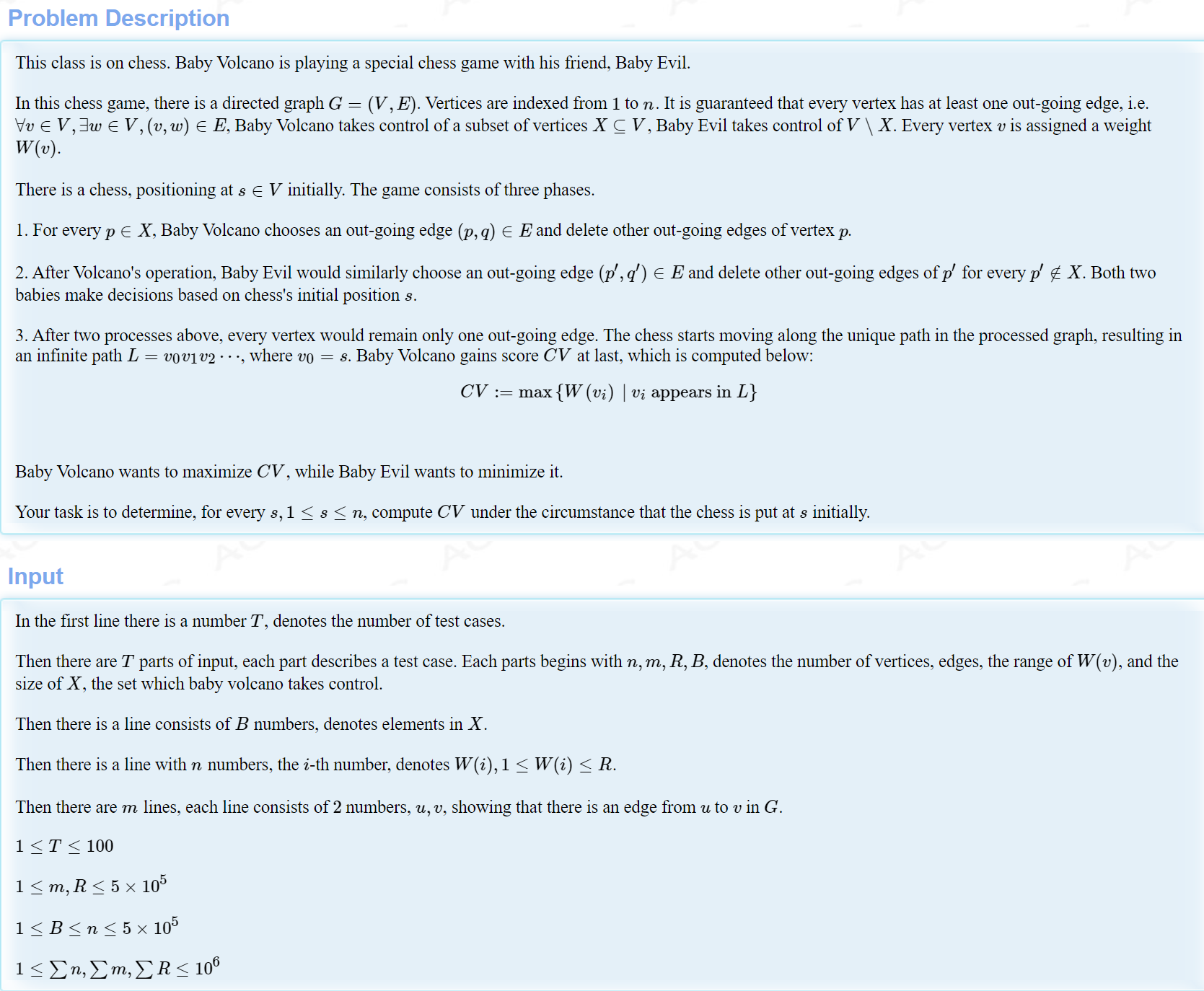# 2020 ccpc 网络赛 1004 Chess Class1 #include<iostream>
2 #include<cstdlib>
3 #include<cstdio>
4 #include<cstring>
5 #include<algorithm>
6 #include<queue>
7 #include<cmath>
8 #define N 500005
9 using namespace std;
10 int T,n,m,R,B;
11 int bj[N];
12 int W[N];
13 int a[N],zz,rd[N];
14 struct ro{
15     int to,next;
17 void build(int x,int y)
18 {
19     zz++;
22     a[x]=zz;
23 }
24 int A[N];
25 bool cmp(int x,int y)
26 {
27     return W[x]>W[y];
28 }
29 queue<int> q1;
30 int ans[N];
31 int cnt;
32 void bfs(int X)
33 {
34     while(!q1.empty())
35     {
36         int x=q1.front();q1.pop();
38         {
40             if(ans[y]!=-1)continue;
41             if(bj[y])
42             {
43                 ans[y]=X;
44                 if(y!=x) q1.push(y);
45             }
46             else
47             {
48                 rd[y]--;
49                 if(!rd[y])
50                 {
51                     ans[y]=X;
52                     if(y!=x) q1.push(y);
53                 }
54             }
55         }
56     }
57 }
58 int main()
59 {
60     scanf("%d",&T);
61     while(T--)
62     {
63         cnt++;
64         scanf("%d%d%d%d",&n,&m,&R,&B);
65         memset(bj,0,sizeof(bj));
66         zz=0;
67         memset(a,0,sizeof(a));
68         memset(rd,0,sizeof(rd));
69         memset(ans,-1,sizeof(ans));
70         for(int i=1;i<=B;i++)
71         {
72             int x;
73             scanf("%d",&x);
74             bj[x]=1;
75         }
76         for(int i=1;i<=n;i++)
77         {
78             scanf("%d",&W[i]);
79             A[i]=i;
80         }
81         for(int i=1;i<=m;i++)
82         {
83             int x,y;
84             scanf("%d%d",&x,&y);
85             build(y,x);
86             rd[x]++;
87         }
88         sort(A+1,A+n+1,cmp);
89         for(int i=1;i<=n;i++)
90         {
91             int j=i;
92             for(;j<=n;j++)
93             {
94                 if(W[A[j]]!=W[A[i]])
95                 {
96                     j--;
97                     break;
98                 }
99             }
100             for(int k=i;k<=j;k++)
101             {
102                 if(ans[A[k]]==-1)
103                 {
104                     q1.push(A[k]);
105                     ans[A[k]]=W[A[i]];
106                 }
107
108             }
109             bfs(W[A[i]]);
110             i=j;
111         }
112         printf("Case #%d:\n",cnt);
113         for(int i=1;i<n;i++) printf("%d ",ans[i]);
114         printf("%d\n",ans[n]);
115     }
116     return 0;
117 }
View Code

posted @ 2020-09-26 10:03  Hzoi_joker  阅读(209)  评论(0编辑  收藏  举报